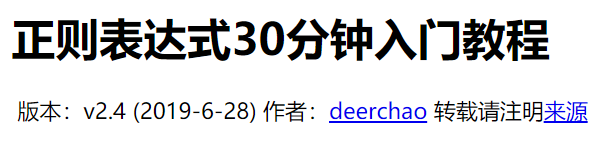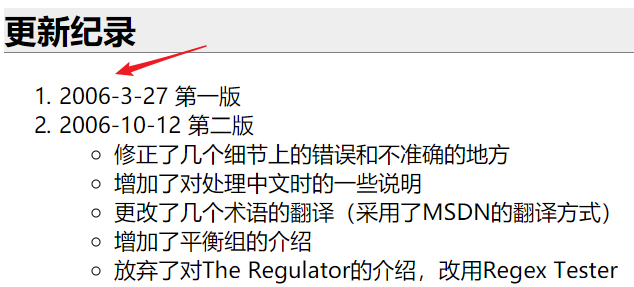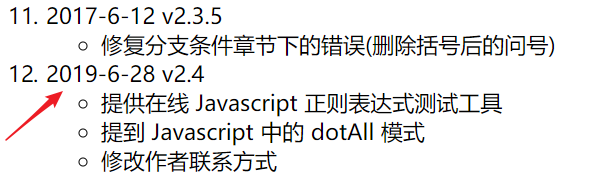# 30分钟的教程写了13年，这回正则表达式有救了!

#### 关于正则表达式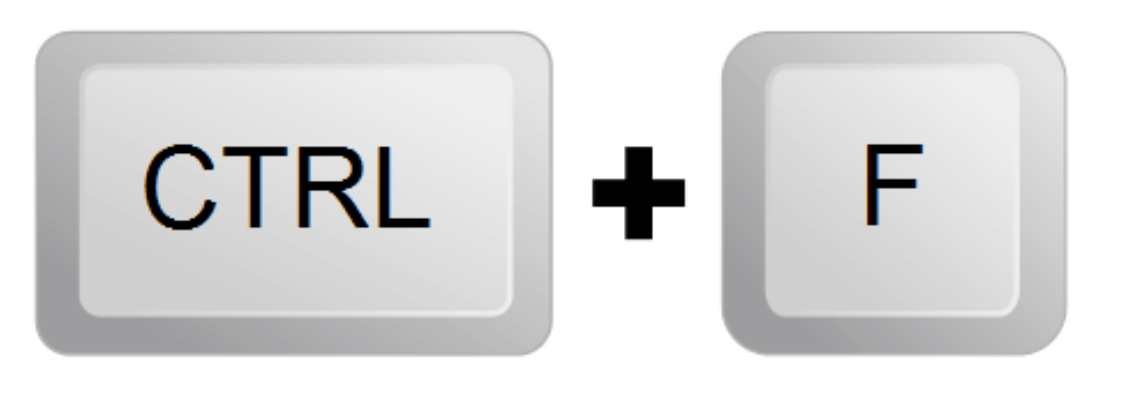``````ATG([ATCG]{3})+(TGA|TAG|TAA)
``````

R语言的正则表达式是让我头疼的，所以我干脆就没有去学。一件事情，只要不是刚需，就有拖延的可能性。后来男神Hadley Wickham 写了一个R包`stringr`使得流程简化，里面规范了正则表达式相关函数的名称。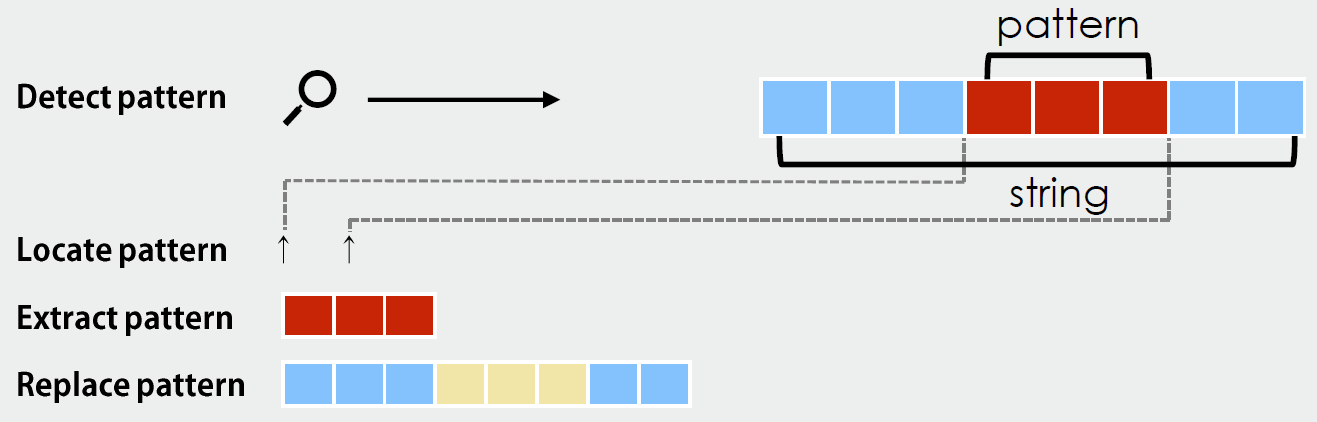1. 查找：Detect pattern，确定这个模式有没有
2. 定位：Locate pattern， 返回模式起止位置
3. 取回：Extract pattern, 返回模式匹配到的条目
4. 替换：Replace pattern，替换匹配的模式，返回替换后的结果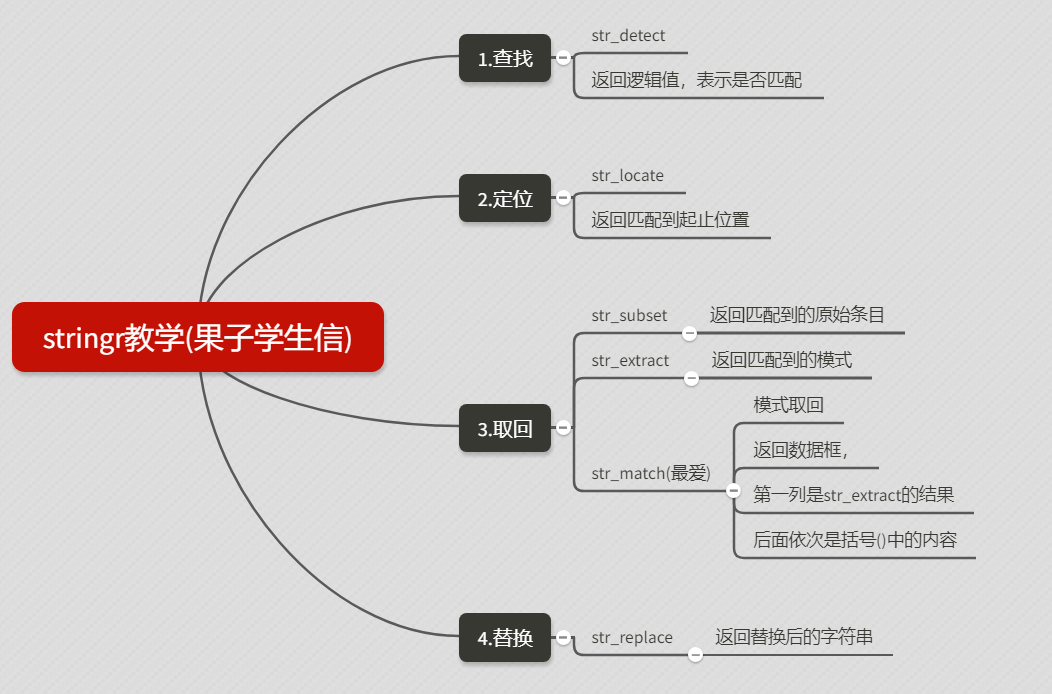#### 学习字符串处理的R包stringr

``````strings <- c(
"apple",
"219 733 8965",
"329-293-8753",
"Work: 579-499-7527; Home: 543.355.3679"
)
``````

#### 1.正则表达式中的三个括号

``````[]
{}
()
``````

#### 2.编写第一个正则表达式

``````pattern <- "([1-9][0-9]{2})[- .]([0-9]{3})[- .]([0-9]{4})"
``````

``````[1-9][0-9]{2}
[0-9]{3}
[0-9]{4}
``````

`[0-9]{3}``[0-9]{4}`分别表示3位数和四位数

``````pattern <- "([1-9][0-9]{2})[- .]([0-9]{3})[- .]([0-9]{4})"
``````

#### 3.用str_view函数学习正则表达式

``````str_view(strings, pattern)
``````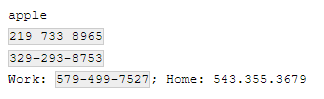1.查找
`str_detect`，从strings中检测能否和pattern匹配，返回的是逻辑值，有点像grepl

``````str_detect(strings, pattern)
``````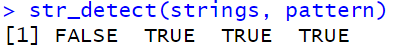2.定位
`str_locate`，从strings中检测能否和pattern匹配，返回匹配的起止位置

``````str_locate(strings, pattern)
``````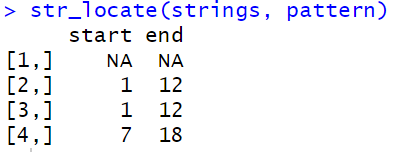3.取回

``````str_subset(strings, pattern)
``````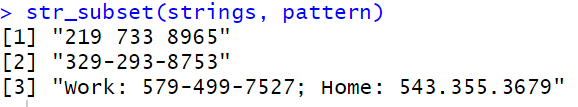``````str_extract(strings, pattern)
``````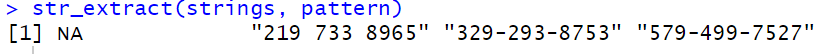``````str_match(strings, pattern)
``````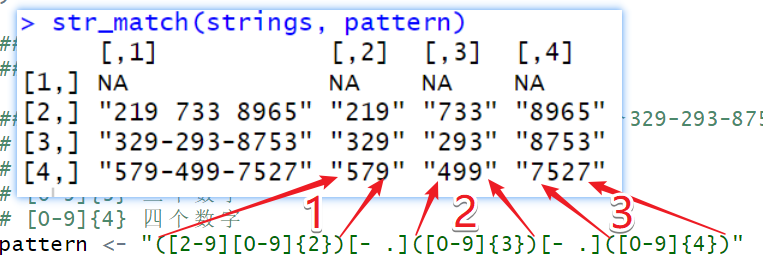4.替换
`str_replace`从strings中能够精确匹配pattern的内容，并替换为''XXX-XXX-XXXX''(此处自定义)

``````str_replace(strings, pattern, "XXX-XXX-XXXX")
``````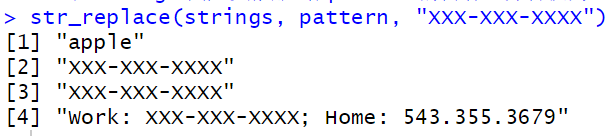#### 4.使用正则表达式来提取GEO基因symbol

(这部分内容以前写过，我复制了过来，复制自己已发布的内容，也算是抄袭)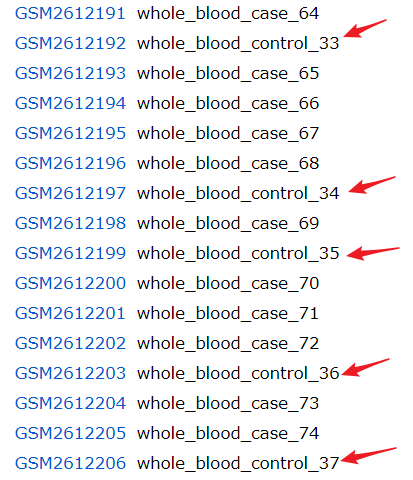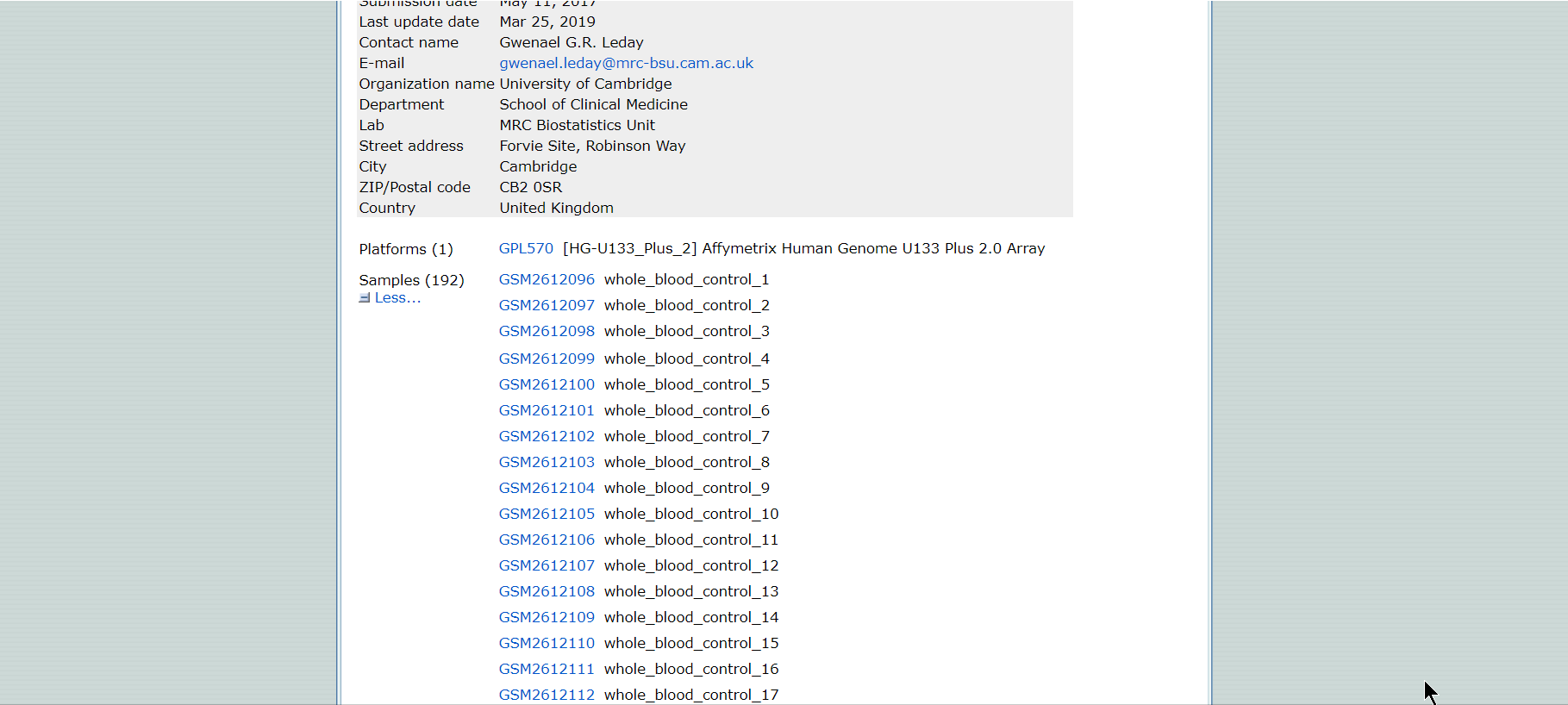``````data <- data.table::fread("gsm_group.txt",data.table = F,header = F)
names(data) <- c("GSM","group")
``````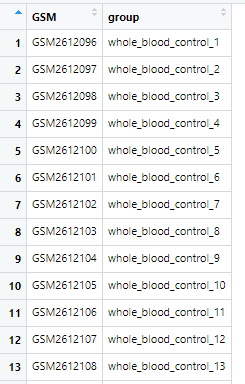``````library(dplyr)
library(tidyr)
separate(group,into = c("drop","group"),sep = "_blood_") %>%
select(-drop) %>%
separate(group,into = c("group","drop"),sep = "_") %>%
select(-drop)
``````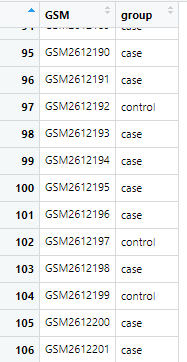``````？
+
*
``````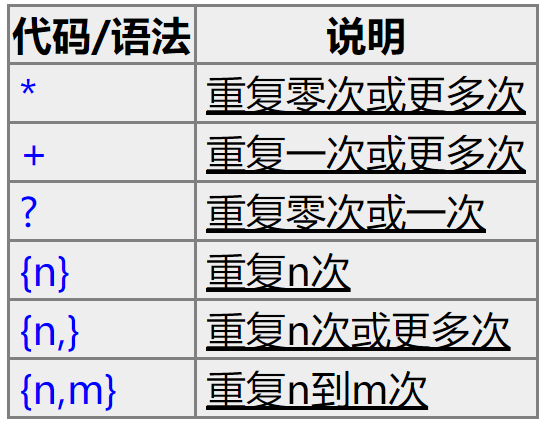``````library(stringr)
group <- str_match(data\$group,"whole_blood_(.*)_.*")
``````

``````whole_blood_(.*)_.*
``````

• 先出现`whole_blood_`
• 再出现一串字符`control`
• 后面跟着下划线`_`
• 最后跟着一串字符结束`33`

• 第一部分是，`whole_blood_`
• 第二部分是任意个数的字符`.*`
• 第三部分是下划线`_`,
• 第四部分是任意个数的字符`.*`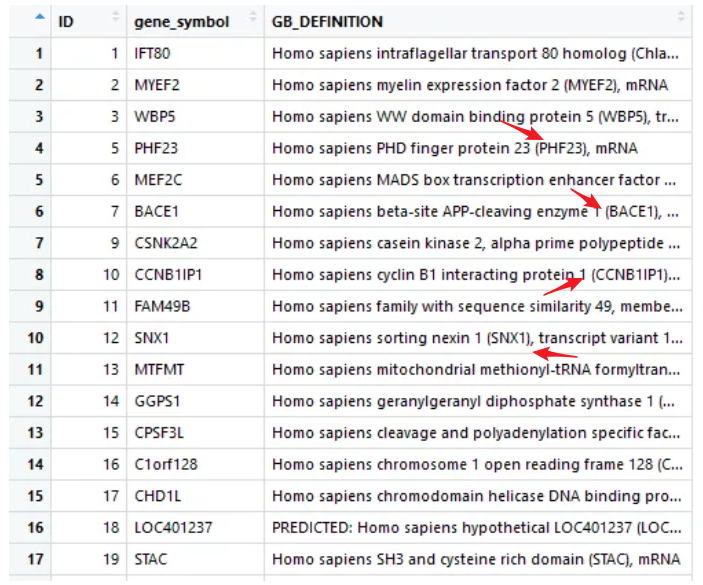#### 5.匹配编码区域CDS

``````ATG([ATCG]{3})+(TGA|TAG|TAA)
``````

`ATG([ATCG]{3})+`

``````ATG([ATCG]{3})+(TGA|TAG|TAA)
``````

#### 6.转义

``````'
*
?
+
|
``````

``````str_split("a.b.c",pattern = ".")
``````

``````[]
 "" "" "" "" "" ""
``````

``````str_split("a.b.c",pattern = "\\.")
``````

``````[]
 "a" "b" "c"
``````

https://cran.r-project.org/web/packages/stringr/vignettes/stringr.html